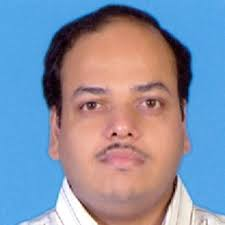# Partial Differential Equations

By Prof. Sivaji Ganesh   |   IIT Bombay
Learners enrolled: 1460
Partial Differential Equations (PDEs) appear as mathematical models for many a physical phenomena. Closed-form solutions to most of these PDEs cannot be found. One of the possible ways to understand the models is by studying the qualitative properties exhibited by their solutions.

In this course, we study first order nonlinear PDEs, and the properties of the three important types of second order linear PDEs (Wave, Laplace, Heat) would be studied and compared.

INTENDED AUDIENCE :Mathematics, Physics, Mechanical Engineering, Chemical Engineering
PRE-REQUISITES   : Exposure to Multivariable calculus is needed.  In addition, exposure to Linear algebra  would be ideal.
INDUSTRY SUPPORT : NILL

Summary
 Course Status : Ongoing Course Type : Core Duration : 12 weeks Start Date : 26 Jul 2021 End Date : 15 Oct 2021 Exam Date : 23 Oct 2021 IST Enrollment Ends : 09 Aug 2021 Category : Mathematics Credit Points : 3 Level : Postgraduate

### Course layout

Week 1:Introduction, First order partial differential equations, Method of characteristics
Week 2:Cauchy problem for Quasilinear first order partial differential equations
Week 3:Cauchy problem for fully nonlinear first order partial differential equations
Week 4:Classification of Second order partial differential equations and Canonical forms
Week 5:Wave equation: d’Alembert’s formula, Solution of wave equation on bounded domains
Week 6:Wave equation: Solution by method of separation of variables, Wave equation in two and three space dimensions
Week 7:Wave equation: Parallelogram identity, Domain of dependence, Domain of influence, Causality principle
Week 8:Wave equation: Finite speed of propagation, Conservation of energy, Huygens principle, Propagation of confined disturbances
Week 9:Laplace equation: Boundary value problems, Fundamental solution, Construction of Greens function for Dirichlet problem posed on special domains.
Week 10:Laplace equation: Poisson’s formula, Solution of Dirichlet problem on a rectangle by method of separation of variables
Week 11:Laplace equation: Mean value property, Maximum principles, Dirichlet principle
Week 12:Heat equation: Fundamental solution, Solution of initial-boundary value problem by separation of variables method, Maximum principle.

### Books and references

1. F. John , Partial differential equations , (Springer, 1982).
2. Y. Pinchover and J. Rubinstein, An introduction to partial differential equations, (Cambridge, 2005).

### Instructor bio### Prof. Sivaji Ganesh

IIT Bombay
I did my M.Sc in Mathematics from University of Hyderabad, and Ph.D from Indian Institute of Science, Bangalore. I worked as a post-doctoral fellow at TIFR Bangalore, University of Joseph Fourier and University of Jean-Monnet before joining Indian Institute of Technology Bombay. My research interests lie in the asymptotic analysis of Partial differential equations.

### Course certificate

The course is free to enroll and learn from. But if you want a certificate, you have to register and write the proctored exam conducted by us in person at any of the designated exam centres.
The exam is optional for a fee of Rs 1000/- (Rupees one thousand only).
Date and Time of Exams: 23 October 2021 Morning session 9am to 12 noon; Afternoon Session 2pm to 5pm.
Registration url: Announcements will be made when the registration form is open for registrations.
The online registration form has to be filled and the certification exam fee needs to be paid. More details will be made available when the exam registration form is published. If there are any changes, it will be mentioned then.
Please check the form for more details on the cities where the exams will be held, the conditions you agree to when you fill the form etc.

CRITERIA TO GET A CERTIFICATE

Average assignment score = 25% of average of best 8 assignments out of the total 12 assignments given in the course.
Exam score = 75% of the proctored certification exam score out of 100

Final score = Average assignment score + Exam score

YOU WILL BE ELIGIBLE FOR A CERTIFICATE ONLY IF AVERAGE ASSIGNMENT SCORE >=10/25 AND EXAM SCORE >= 30/75. If one of the 2 criteria is not met, you will not get the certificate even if the Final score >= 40/100.

Certificate will have your name, photograph and the score in the final exam with the breakup.It will have the logos of NPTEL and IIT Bombay.It will be e-verifiable at nptel.ac.in/noc.

Only the e-certificate will be made available. Hard copies will not be dispatched.

Once again, thanks for your interest in our online courses and certification. Happy learning.

- NPTEL team